Search

# Sigmoid Neuron — Building Block of Deep Neural Networks

The building block of the deep neural networks is called the sigmoid neuron. Sigmoid neurons are similar to perceptrons, but they are slightly modified such that the output from the sigmoid neuron is much smoother than the step functional output from perceptron. In this post, we will talk about the motivation behind the creation of sigmoid neuron and working of the sigmoid neuron model.

This is the 1st part in the two-part series discussing the working of sigmoid neuron and it’s learning algorithm:

1 | Sigmoid Neuron — Building Block of Deep Neural Networks

Why Sigmoid Neuron?

Before we go into the working of a sigmoid neuron, let's talk about the perceptron model and its limitations in brief.

Perceptron model takes several real-valued inputs and gives a single binary output. In the perceptron model, every input xi has weight wi associated with it. The weights indicate the importance of the input in the decision-making process. The model output is decided by a threshold Wₒ if the weighted sum of the inputs is greater than threshold Wₒ output will be 1 else output will be 0. In other words, the model will fire if the weighted sum is greater than the threshold.

Perceptron (Left) & Mathematical Representation (Right)From the mathematical representation, we might say that the thresholding logic used by the perceptron is very harsh. Let’s see the harsh thresholding logic with an example. Consider the decision making process of a person, whether he/she would like to purchase a car or not based on only one input X1 — Salary and by setting the threshold b(Wₒ) = -10 and the weight W = 0.2. The output from the perceptron model will look like in the figure shown below.Data (Left) & Graphical Representation of Output(Right)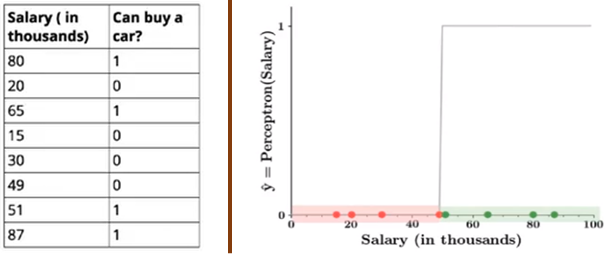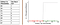Red points indicates that a person would not buy a car and green points indicate that person would like to buy a car. Isn’t it a bit odd that a person with 50.1K will buy a car but someone with a 49.9K will not buy a car? The small change in the input to a perceptron can sometimes cause the output to completely flip, say from 0 to 1. This behavior is not a characteristic of the specific problem we choose or the specific weight and the threshold we choose. It is a characteristic of the perceptron neuron itself which behaves like a step function. We can overcome this problem by introducing a new type of artificial neuron called a sigmoid neuron. To know more about the working of the perceptron, kindly refer to my previous post on the Perceptron Model

1. Sigmoid Neuron Can we have a smoother (not so harsh) function?Introducing sigmoid neurons where the output function is much smoother than the step function. In the sigmoid neuron, a small change in the input only causes a small change in the output as opposed to the stepped output. There are many functions with the characteristic of an “S shaped curve known as sigmoid functions. The most commonly used function is the logistic function.Sigmoid Neuron Representation (logistic function)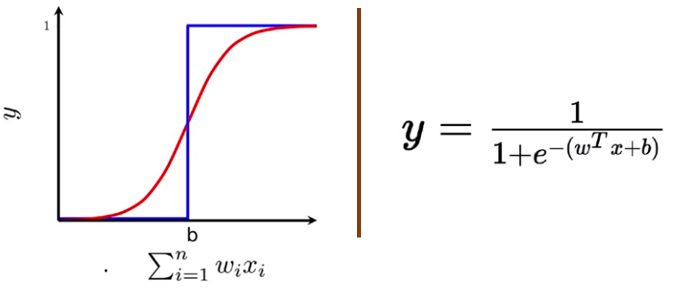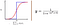We no longer see a sharp transition at the threshold b. The output from the sigmoid neuron is not 0 or 1. Instead, it is a real value between 0–1 which can be interpreted as a probability.Data & Task Regression and ClassificationThe inputs to the sigmoid neuron can be real numbers unlike the boolean inputs in MP Neuron and the output will also be a real number between 0–1. In the sigmoid neuron, we are trying to regress the relationship between X and Y in terms of probability. Even though the output is between 0–1, we can still use the sigmoid function for binary classification tasks by choosing some threshold.Learning Algorithm In this section, we will discuss an algorithm for learning the parameters w and b of the sigmoid neuron model by using the gradient descent algorithm.Minimize the Squared Error Loss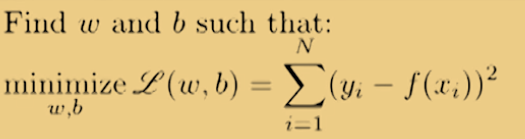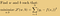The objective of the learning algorithm is to determine the best possible values for the parameters, such that the overall loss (squared error loss) of the model is minimized as much as possible. Here goes the learning algorithm:Sigmoid Learning Algorithm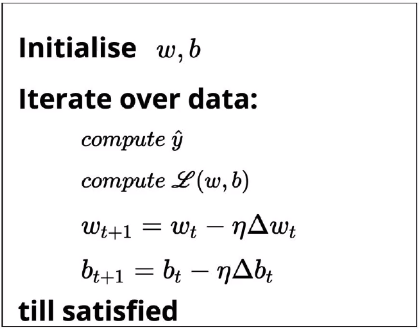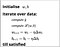We initialize w and b randomly. We then iterate over all the observations in the data, for each observation find the corresponding predicted outcome using the sigmoid function and compute the squared error loss. Based on the loss value, we will update the weights such that the overall loss of the model at the new parameters will be less than the current loss of the model.Loss Optimization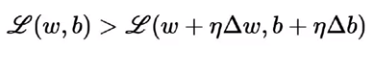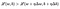We will keep doing the update operation until we are satisfied. Till satisfied could mean any of the following:The overall loss of the model becomes zero.The overall loss of the model becomes a very small value closer to zero.Iterating for a fixed number of passes based on computational capacity.Can It Handle Non-Linear Data? One of the limitations of the perceptron model is that the learning algorithm works only if the data is linearly separable. That means that the positive points will lie on one side of the boundary and negative points lie another side of the boundary. Can sigmoid neuron handle non-linearly separable data?.Let's take an example of whether a person is going to buy a car or not based on two inputs, X₁ — Salary in Lakhs Per Annum (LPA) and X₂ — Size of the family. I am assuming that there is a relationship between X and Y, it is approximated using the sigmoid function.Input Data(Left) & Scatter Plot of Data(Right)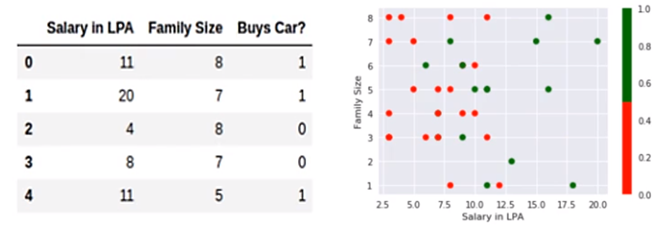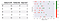The red points indicate that the output is 0 and green points indicate that it is 1. As we can see from the figure, there is no line or a linear boundary that can effectively separate red and green points. If we train a perceptron on this data, the learning algorithm will never converge because the data is not linearly separable. Instead of going for convergence, I will run the model for a certain number of iterations so that the errors will be minimized as much as possible.Perceptron Decision boundary for fixed iterations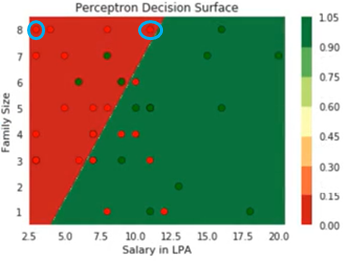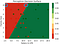From the perceptron decision boundary, we can see that the perceptron doesn’t distinguish between the points that lie close to the boundary and the points lie far inside because of the harsh thresholding logic. But in the real world scenario, we would expect a person who is sitting on the fence of the boundary can go either way, unlike the person who is way inside from the decision boundary.Let’s see how sigmoid neuron will handle this non-linearly separable data. Once I fit our two-dimensional data using the sigmoid neuron, I will be able to generate the 3D contour plot shown below to represent the decision boundary for all the observations.Sigmoid Neuron Decision Boundary (Left) & Top View of Decision Boundary (Right)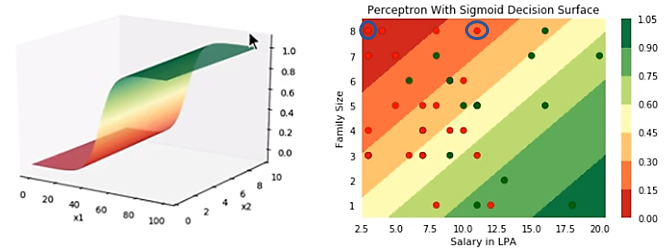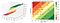For comparison, let’s take the same two observations and see what will be predicted outcome from the sigmoid neuron for these observations. As you can see the predicted value for the observation present in the far left of the plot is zero (present in the dark red region) and the predicted value of another observation is around 0.35 i.e. there is a 35% chance that the person might buy a car. Unlike the rigid output from the perceptron, now we a smooth and continuous output between 0–1 which can be interpreted as a probability. Still does not completely solve our problem for non-linear data.Although we have introduced the non-linear sigmoid neuron function, it is still not able to effectively separate red points from green points. The important point is that from a rigid decision boundary in perceptron, we have taken our first step in the direction of creating a decision boundary that works well for non-linearly separable data. Hence the sigmoid neuron is the building block of deep neural network eventually we have to use a network of neurons to helps us out to create a “perfect” decision boundary.Continue Learning If you are interested in learning more about Artificial Neural Network, check out the Artificial Neural Networks by Abhishek and Pukhraj from Starttechacademy. Also, the course is taught in the latest version of Tensorflow 2.0 (Keras backend). They also have a very good bundle on machine learning (Basics + Advanced) in both Python and R languages.Conclusion In this post, we saw the limitations of the perceptron that led to the creation of sigmoid neuron. We also saw the working of the sigmoid neuron with an example and how it is able to overcome some of the limitations. We have seen how the perceptron and sigmoid neuron models are handling the non-linearly separable data.